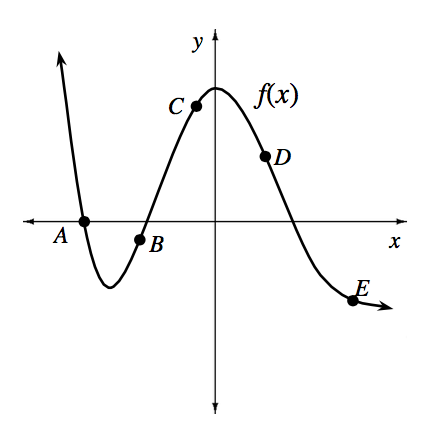### Home > APCALC > Chapter 8 > Lesson 8.1.6 > Problem8-64

8-64.

At which point(s) on the graph of $y = f(x)$ at right, do the following situations occur?

1. $f^\prime(x)$ and $f^{\prime\prime}(x)$ are both positive

Translation: slope of $f\left(x\right)$ is positive AND $f\left(x\right)$ is concave up.

2. $f^\prime(x)$ and $f^{\prime\prime}(x)$ are both negative

Refer to the hint in part (a).

3. $f^\prime(x)$ is positive and $f^{\prime\prime}(x)$ is negative

Refer to the hint in part (a).

4. $f^\prime(x)$ is negative and $f^{\prime\prime}(x)$ is positive

Points $e$ and $a$.